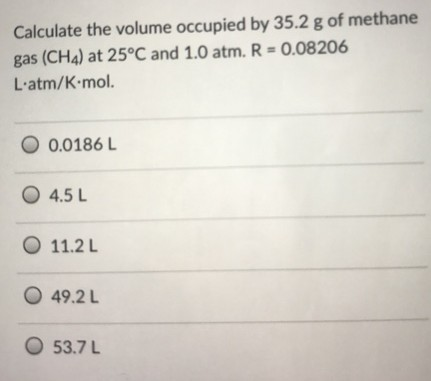# Problem: Calculate the volume occupied by 35.2 g of methane gas (CH4) at 25 ºC and 1.0 atm. R = 0.082060.0186 L. 4.5 L 11.2 L 49.2 L 53.7 L

###### FREE Expert Solution
94% (284 ratings)###### Problem Details

Calculate the volume occupied by 35.2 g of methane gas (CH4) at 25 ºC and 1.0 atm. R = 0.08206
0.0186 L. 4.5 L 11.2 L 49.2 L 53.7 LFrequently Asked Questions

What scientific concept do you need to know in order to solve this problem?

Our tutors have indicated that to solve this problem you will need to apply the The Ideal Gas Law concept. You can view video lessons to learn The Ideal Gas Law. Or if you need more The Ideal Gas Law practice, you can also practice The Ideal Gas Law practice problems.

What professor is this problem relevant for?

Based on our data, we think this problem is relevant for Professor Swamy's class at FIU.